# Methods and formulas for ANOVA table in Nested Gage R&R Study

Select the method or formula of your choice.

## Sum of squares

The sum of squares (SS) is the sum of squared distances, and is a measure of the variability that is from different sources.

SSOperator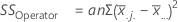TermDescription
anumber of parts
nnumber of replicates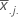mean for each operator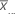grand mean
SSPart (Operator)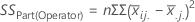TermDescription
nnumber of replicates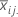mean for each part, i, within operator, jmean for operator j
SSRepeatability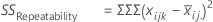TermDescription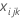each observationmean for each part, i, within operator, j
SSTotal
SSTotal = SSOperator + SSPart (Operator) + SSRepeatability

## Degrees of freedom

The degrees of freedom (DF) for each SS (sums of squares). In general, DF measures how much information is available to calculate each SS.

DFOperator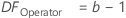TermDescription
bnumber of operators
DFPart (Operator)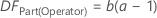TermDescription
anumber of parts measured by each operator
bnumber of operators
DFRepeatability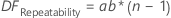TermDescription
anumber of parts
bnumber of operators
nnumber of replicates
DFTotal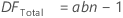TermDescription
anumber of parts
bnumber of operators
nnumber of replicates

## Mean squares

The mean squares (MS) is the variability in the data from different sources. MS accounts for the fact that different sources have different numbers of levels or possible values.

MSOperator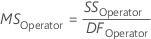MS Part (Operator)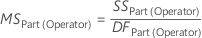MS Repeatability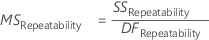## F

The F-statistic is used to determine whether the effects of Operator or Part (Operator) significantly impact the measurement.

FOperator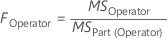FPart(Operator)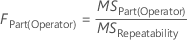## p-value

The p-value is the probability of obtaining a test statistic (such as the F-statistic) that is at least as extreme as the value that is calculated from the sample, if the null hypothesis is true.

By using this site you agree to the use of cookies for analytics and personalized content.  Read our policy